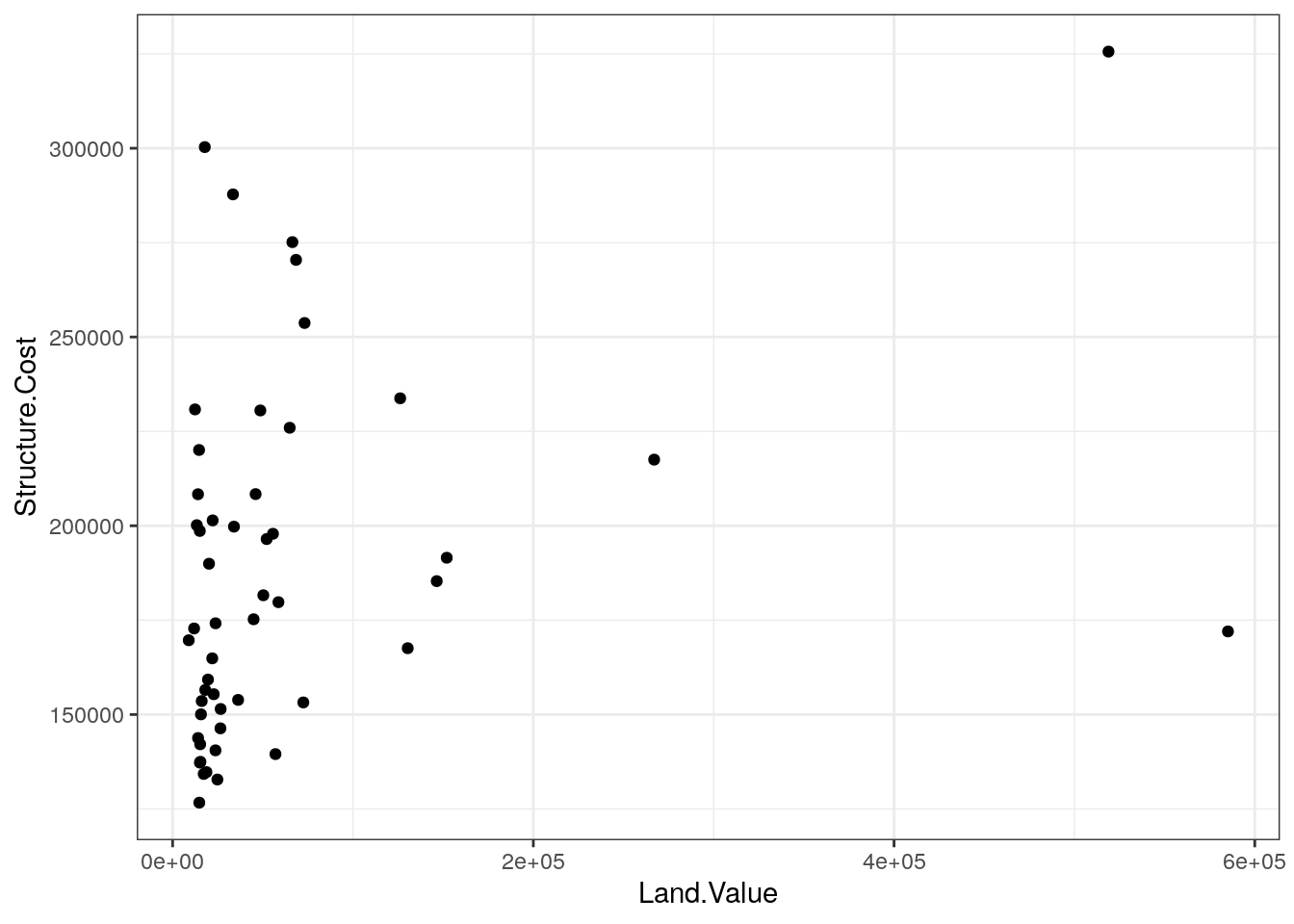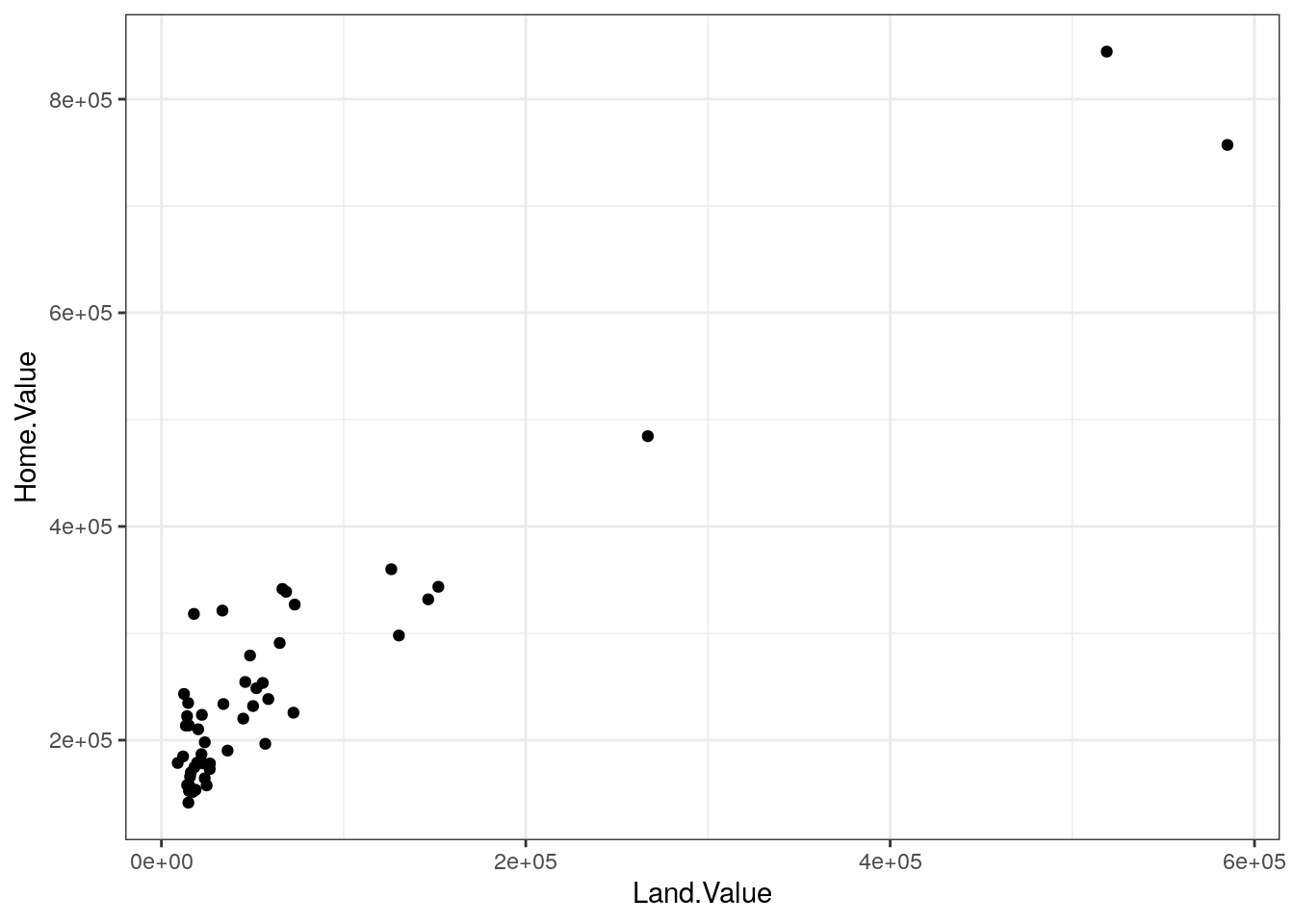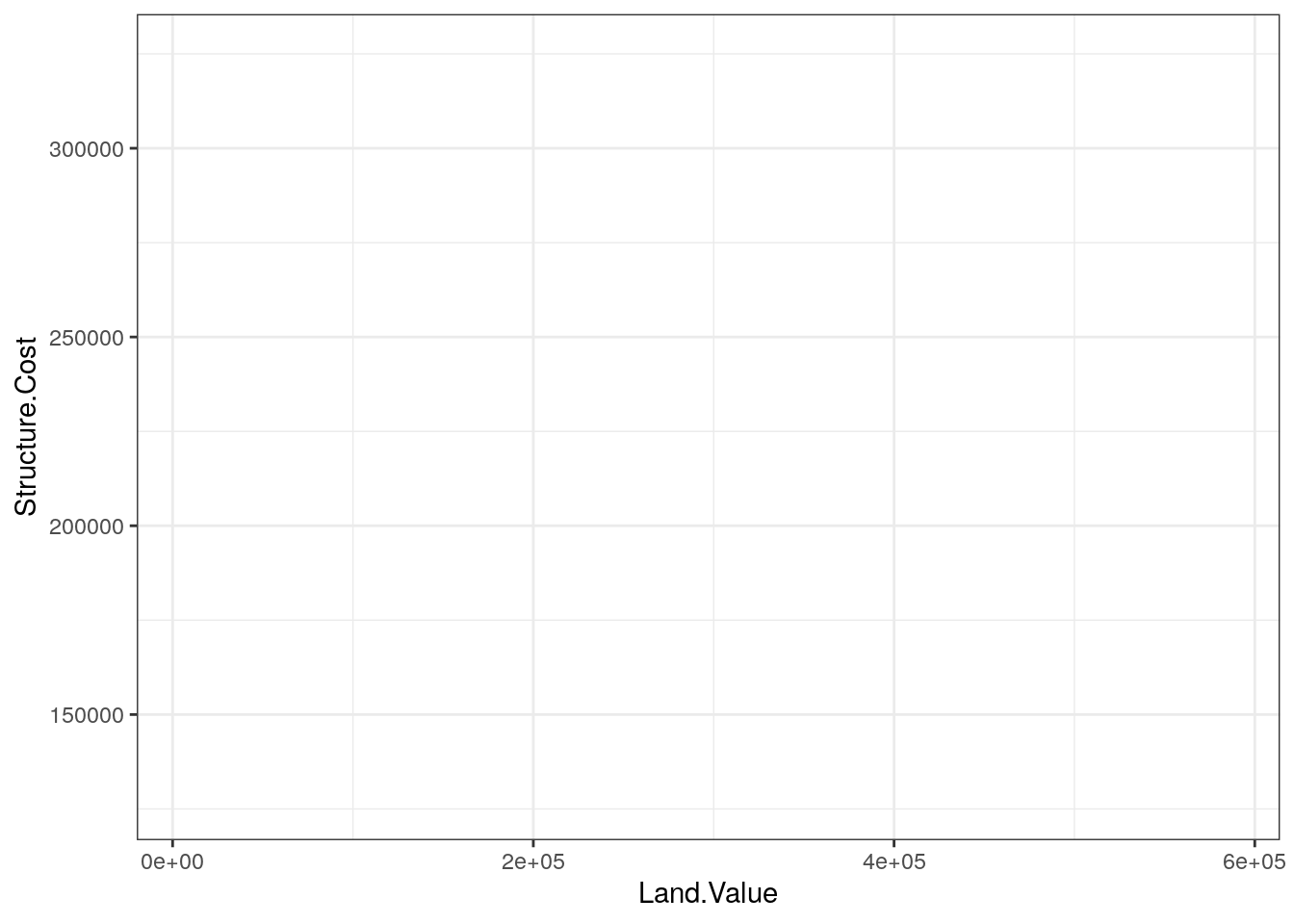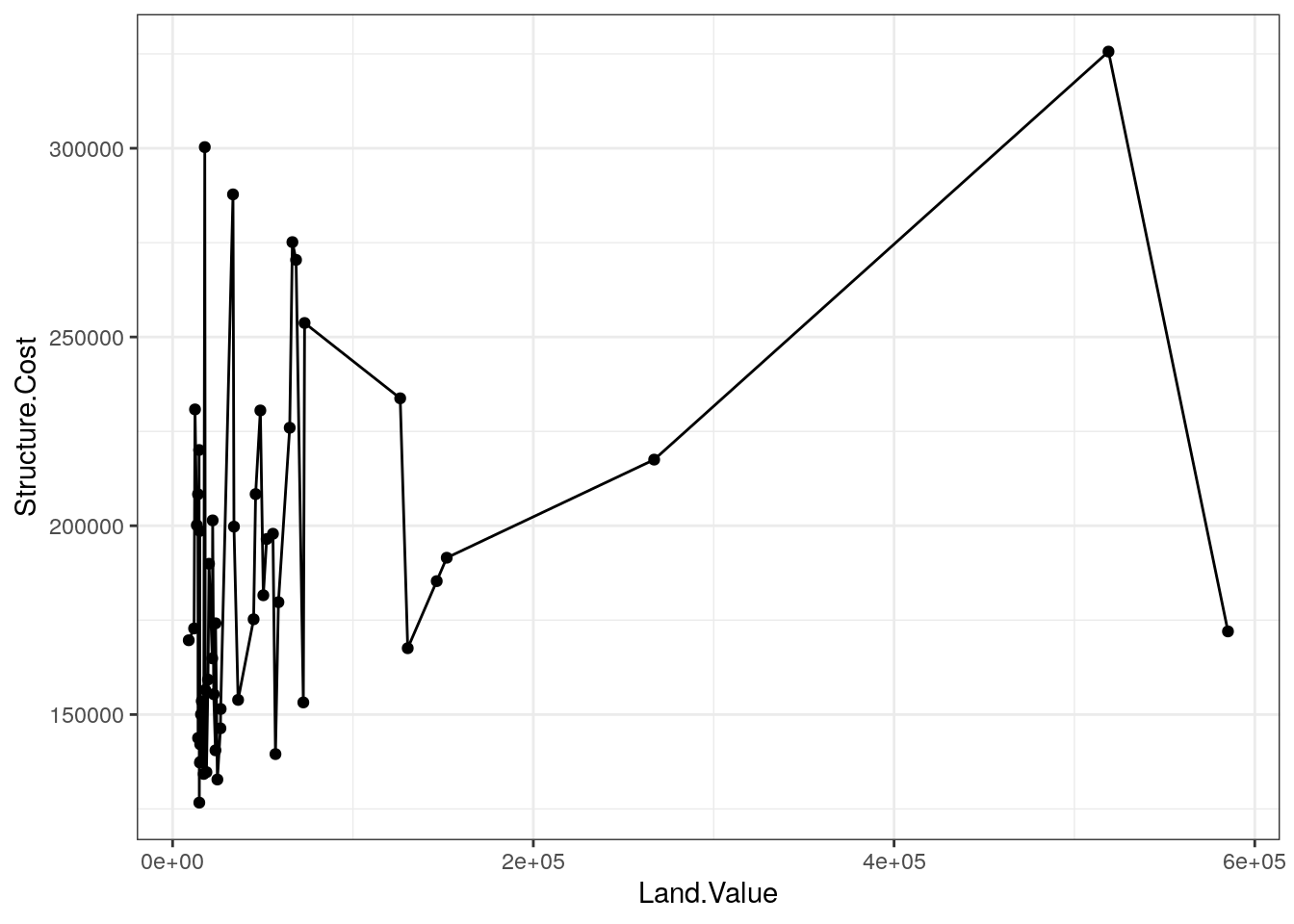## Before You Start

• Make a new RStudio project for Lab 3, and put it in a sensible place within your `stat209` folder.
• Save a copy of this `.Rmd` file (use Save As…) in that project folder
• Fill in your name in the author field, and today’s date in the Date.
• Before doing anything else, save the file, and make sure you can Knit it

## RMarkdown Reference

• Bold text with two *s on either side
• Italicized text with _ on either side
• Create blocks of R code with Insert > R (or use a keyboard shortcut). Chunks begin and end with a line containing three backticks (on the tilde key on most keyboards).
• Lines between the ``` lines are interpreted as code
• Can run individual chunks, or all chunks prior to a chunk, using the buttons in the top right of the chunk region
• “Compile” the document to HTML with “Knit > Knit to HTML”
• The opening line of a code chunk has curly braces with some chunk options separated by commas.
• Use `message = FALSE` in the braces to suppress info from R as when loading packages
• Use `warning = FALSE` if you are getting a warning that you’re convinced isn’t a problem, and you don’t want it to be displayed (but be sure first!)
• Use `echo = FALSE` if you don’t want the code to show up in the output
• Use `eval = FALSE` if you want the code to be displayed but not run
• Use `results = 'hide'` if you want the code to be run but don’t want the results to be displayed
• Use `cache = TRUE` for time-consuming chunks so they don’t re-run every time

### Reminder

With Markdown documents: When Knitting the entire document (unlike when running individual chunks), the process does not have access to objects in your environment: only objects defined within the document are available.

This is useful, since if you left something undefined you’ll usually get an error. But you will not always want to Knit the entire document, since this can take time; be careful when running one chunk at a time, since this can use objects in your environment.

## Graphics with `ggplot2`

Goal: By the end of this lab, you will be able to use `ggplot2` to build some basic data graphics

### Setting up

Before we can use a library like `ggplot2`, we have to load it. In this case, we load the `tidyverse` package, which automatically loads `ggplot2` for us (since it depends on it).

1. Make a new code chunk to load the `tidyverse` package using the `library()` function. Adjust the chunk options to suppress `message`s. Knit the document.

Note: Remember, you shouldn’t copy and paste code directly from the web. Type it out yourself so that you slow yourself down a bit to process what you’re reading, and to develop your muscle memory.

##### Solution

Click the “Code” button on the Knitted version of this lab on the course website to see my solution, but not until after you’ve written yours!

``library(tidyverse)``

### Why `ggplot2`?

Advantages of `ggplot2`:

• Consistent underlying grammar of graphics (Wilkinson, 2005)
• Is a mature and complete graphics system
• Plot specification is at a high level of abstraction
• Flexible
• Has a `theme` system to polish plot appearance (more on this later)
• Used by many, many people

### What is The Grammar Of Graphics?

The big idea: independenly specify plot building blocks and combine them to create just about any kind of graphical display you want. Building blocks of a graph include:

• data (`data=`)
• aesthetic mappings (`aes()`)
• geometric objects (`geom_*()`)
• statistical transformations
• scales
• coordinate systems
• faceting

Using `ggplot2`, we can specify different parts of the plot, and combine them together using the `+` operator.

### Example: Housing prices

Let’s start by looking at some data on housing prices:

``````housing <- read_csv("http://colindawson.net/data/landdata-states.csv")
glimpse(housing)``````
``````## Rows: 7,803
## Columns: 11
## \$ State            <chr> "AK", "AK", "AK", "AK", "AK", "AK", "AK", "AK", …
## \$ region           <chr> "West", "West", "West", "West", "West", "West", …
## \$ Date             <dbl> 2010.25, 2010.50, 2009.75, 2010.00, 2008.00, 200…
## \$ Home.Value       <dbl> 224952, 225511, 225820, 224994, 234590, 233714, …
## \$ Structure.Cost   <dbl> 160599, 160252, 163791, 161787, 155400, 157458, …
## \$ Land.Value       <dbl> 64352, 65259, 62029, 63207, 79190, 76256, 72906,…
## \$ Land.Share..Pct. <dbl> 28.6, 28.9, 27.5, 28.1, 33.8, 32.6, 31.3, 29.9, …
## \$ Home.Price.Index <dbl> 1.481, 1.484, 1.486, 1.481, 1.544, 1.538, 1.534,…
## \$ Land.Price.Index <dbl> 1.552, 1.576, 1.494, 1.524, 1.885, 1.817, 1.740,…
## \$ Year             <dbl> 2010, 2010, 2009, 2009, 2007, 2008, 2008, 2008, …
## \$ Qrtr             <dbl> 1, 2, 3, 4, 4, 1, 2, 3, 4, 1, 2, 2, 3, 4, 1, 2, …``````

(Data originally from https://www.lincolninst.edu/subcenters/land-values/land-prices-by-state.asp, via Jordan Crouser at Smith College)

## Geometric Objects and Aesthetics

### Geometric Objects (`geom`)

Geometric objects or `geoms` are the actual marks we put on a plot. Examples include:

• points (`geom_point()`, for scatter plots, dot plots, etc.)
• lines (`geom_line()`, for time series, trend lines, etc.)
• boxplot (`geom_boxplot()`, for, um…)

among others

A plot must have at least one `geom`, but you can combine multiple `geom`s in a single plot. Remember that you can add elements to an existing plot using the `+` operator (elements can be chained together in a single command, or intermediate plots can be assigned to a variable and added to later).

You can see a list of the `geom_*()` functions in `ggplot2` using the following command:

``help.search("geom_", package = "ggplot2")``

In RStudio, if you simply type `geom_` and then press the tab key, you will see a dropdown list of possible ways to complete the text. This is a useful trick generally, to save repetitive typing. Once you have completed a function name and typed the open paren `(`, tab will also show you a list of valid argument names for that function.

### Aesthetic Mappings (`aes`)

In `ggplot2`, aesthetic means “something you can see”. Each aesthetic is a mapping between a visual cue and a variable. For example, we can map variables to the following cues:

• position (i.e., on the x and y axes)
• color (the “outside” color of a geometric object)
• fill (the “inside” color of a geometric object)
• shape (of points)
• line type
• size

Each type of `geom` accepts only a subset of all aesthetics — refer to the help pages of individual `geom_()` functions to see what mappings each `geom` accepts. Aesthetic mappings are set with the `aes()` function.

### Points

Now that we know about geometric objects and aesthetic mapping, we’re ready to make out first `ggplot`: a scatterplot. We’ll use `geom_point` to do this, which requires `aes` mappings for `x` and `y`. Other mappings (such as color) are optional.

#### EXAMPLE

``````## Get a subset of the data (more on filter() later)
hp2013Q1 <- filter(housing, Date == 2013.25)

ggplot(hp2013Q1, aes(y = Structure.Cost, x = Land.Value)) +
geom_point()``````### The “pipe” (`%>%`) operator

In the `filter()` command above, the function `filter` takes the existing dataset called `housing`, and extracts only those cases where the entry in the `Date` column is equal to `"2013.25"`, returning the result in a new dataset object, which we give the label `hp2013Q1`.

The function took two arguments: the dataset, and a logical condition that serves as the “filter”; only letting through cases that meet a certain criterion, and returned a dataset.

Often times we will perform operations like this, which take a dataset as an argument, and return a modified dataset, in sequence, “chaining” them together. One of the packages in the `tidyverse` augments the R language itself with an additional operator called the pipe operator (written as `%>%`).

``filter(housing, Date == 2013.25)``

as we did above, we can instead “pipe” the data into the filter, writing the command as follows.

``housing %>% filter(Date == 2013.25)``

What’s happening here is the `housing` dataset is “fed through the pipe”, and passed on to the `filter()` function as its first argument. This whole expression then returns the 2013 Q1 subset of the data.

1. Create a scatterplot of the value of each home in the first quarter of 2013 as a function of the value of the land. Try to write the plot command using the pipe operator to pass the data to the `ggplot` function.

##### Possible Solution
``````## The line breaks and indentation in this code don't change the meaning of the command, but it
## makes code easier to read if each element is on its own line
hp2013Q1 %>%
ggplot(aes(y = Home.Value, x = Land.Value)) +
geom_point()``````### Plot objects

The output of the `ggplot()` function is an object. Since we want to modify the plot that we created above, it’s helpful to store the plot object in a named variable.

``````base_plot <-
hp2013Q1 %>%
ggplot(aes(y = Structure.Cost, x = Land.Value))``````

To actually show the plot, we just print it, as we would print the value of a numeric value or a data frame.

``base_plot``Notice that although the axes are set up and labeled, there’s no data being depicted. That’s because we haven’t specified any `geom`s – in other words, we haven’t told R what we actually want it to draw. However, the aesthetic mapping is defined, and if we take this base plot and add `geom`s to it, the resulting plots will use the mapping that we defined in `base_plot`.

``base_plot + geom_point()``1. We have a lovely scatterplot now, but we haven’t stored it. Store the scatterplot you created in the previous exercise as an object called `home_value_plot`.

##### Possible Solution
``````home_value_plot <-
hp2013Q1 %>%
ggplot(aes(y = Home.Value, x = Land.Value)) +
geom_point()``````

### Lines

A plot constructed with `ggplot` can have more than one `geom`. For example, we could connect all of the points using `geom_line()`. By default, the aesthetic mapping defined in the base plot is carried over to any new `geom`s that we add. Note that now we see both points and lines.

``base_plot + geom_point() + geom_line()``1. Does it make sense to connect the observations with `geom_line()` in this case? Do the lines help us understand the connections between the observations? What do the lines represent?

##### Response

(this one’s text, not code)

### Smoothers

Not all geometric objects are simple shapes – `geom_smooth()` includes both a line and a “ribbon”, where the line is a “smoothed” moving average of the y variable, and the band is a 95% confidence band, represent our uncertainty about what the moving average actually would be if we had infinite data.

``````base_plot +
geom_point() +
geom_smooth()``````